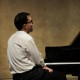# One mathematical problem in combinatorical topology: my lecture at the conference Group 32 in Prague

We formulate one mathematical problem, which will be useful in Quantum Gravity problematics. We will call, that a circle with ﬁnite length and ﬁnite circumference, which could be deformed, is a ring: let’s have a ﬁnite collection of N rings, which could not touch. Derive a formula for number of non-homeomorphic structures, which could be constructed from this finite collection of rings; Every two rings could be linked only once, they could not be knotted or twisted. We don’t consider the Brunnian type of ring.